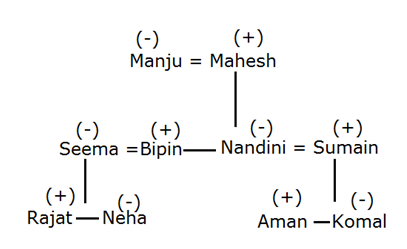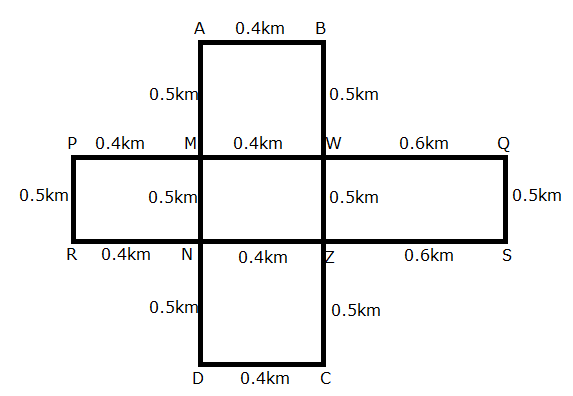# RRB Clerk Mains Reasoning (Day-07)

## Reasoning Materials for RRB Clerk Prelims:

Dear Aspirants, Our IBPS Guide team is providing new series of Reasoning Questions for RRB Clerk Mains 2020 so the aspirants can practice it on a daily basis. These questions are framed by our skilled experts after understanding your needs thoroughly. Aspirants can practice these new series questions daily to familiarize with the exact exam pattern and make your preparation effective.

Start Quiz

Number series- conditions based

Direction (1-3): Two rows of numbers are given. The resultant number in each row is to be worked out separately based on the following rules and the question below the rows of numbers is to be answered. The operations of numbers progress from left to right.

Step I: If an odd number is followed by an even number then the resultant will be the addition of both the numbers.

Step II: If an even number is followed by a perfect square then the resultant will be the subtraction of the square number from the even number.

Step III: If an odd number is followed by another odd number then the resultant will be the addition of both the numbers.

Step IV: If an even number is followed by even number then the resultant comes by multiplying the number.

Note: If two conditions apply for the same number then the step which comes first as in ascending order is only considered. (If 48 and 16 ->  Step II and Step IV followed for this number so, we can take only step II)

1) Find the sum of the resultants of the two rows.

21        5          9

8          6          16

A.30

B.33

C.49

D.16

E.None of these

2) Find the sum of the resultants of the two rows, If X is the resultant of the second row.

X         25        2

3          7          6

A.32

B.57

C.48

D.40

E.97

3) Find the difference between the resultant of the first and the second row.

16        2          25

5          8          9

A.17

B.39

C.20

D.15

E.None of these

Blood relation

Direction (4-5): Study the below details and answer the following questions.

There are ten members in a family – Manju, Mahesh, Bipin, Seema, Nandini, Sumain, Rajat, Neha, Komal and Aman. It is a three generations family and there are three couples in the family.

Manju is Rajat’s grandmother. Sumain is Mahesh’s son in law. Mahesh is a male member in the family. Bipin is the husband of Seema. Nandini is the daughter of Mahesh and has two children, a brother, a niece and a nephew. Rajat is the brother of Neha who is a female. Komal is the sister of Aman who is the son of Nandini.

4) What is the relationship between Mahesh and Bipin?

A.Father and son

B.Father and daughter

C.Brothers

D.Cousins

E.Grandfather and Grandson

5) Who among the following person is the niece of Nandini?

A.Komal

B.Neha

C.Seema

D.Manju

E.Rajat

Direction sense

Direction (6-10): Study the following information carefully and answer the given questions below.

P, Q, R and S are the corners of a rectangular field such that P is to the North of R. Q is 1.4km to the East of P. The smaller side of the rectangular field is 0.5km. A, B, C and D are the corners of another rectangular field such that A is 0.4km to the west of B, which is 1.5km to the north of C.

Rectangle field PQRS intersects the line joining AD and BC at MN and WZ respectively. It is also known that Q is 0.6km towards the East of W and N is 0.5km towards the North of D.

6) If the person “X” wants to reach point B from Point R, then which of the following will be the correct direction to reach his destination?

A.X walks 1000m east from point R, then he takes left turn and walks 500m to reach point B

B.X walks 500m north from point R, then he takes right turn and walks 800m and then he takes left turn and walk 500m and to reach point B.

C.X walks 400m east from point R and then he takes left turn and walks 500m and then he takes right turn and walks 800m to reach point B.

D.X walks 400m east from point R and then he takes a right turn and walks 1000m and then he takes left turn and walk 400m to reach point B.

E.None of these

7) What will be the minimum possible distance to reach point A from point Z?

A.ZSQWMA

B.ZNRPMA

C.ZCDNMA

D.ZWBA

E.None of these

8) What is the position of point Z with respect to point P?

A.North-East

B.South-East

C.North

D.South-West

E.North-West

9) What is the position of point R with respect to point W?

A.North-East

B.South-East

C.North-West

D.South-West

E.None of these

10) What is the position of point Z with respect to point R?

A.North-East

B.East

C.West

D.South-West

E.None of these

Directions (1-5):

1st row step III applied 21+5=26; Step II applied 26-9=17

2nd row step IV applied 8×6=48; step II => 48-16=32

Sum of resultant is 32+17=49

2nd row step III 3+7=10 step IV => 10×6=60

1st row Step II 60-25=35; Step I => 35+2=37

Sum of two rows are 60+37 =97

1st row step IV => 16×2=32, step II => 32-25=7

2nd row step I => 5+8=13, step III => 13+9=22

Difference is 22-7=15

Directions (4-5):Directions (6-10):